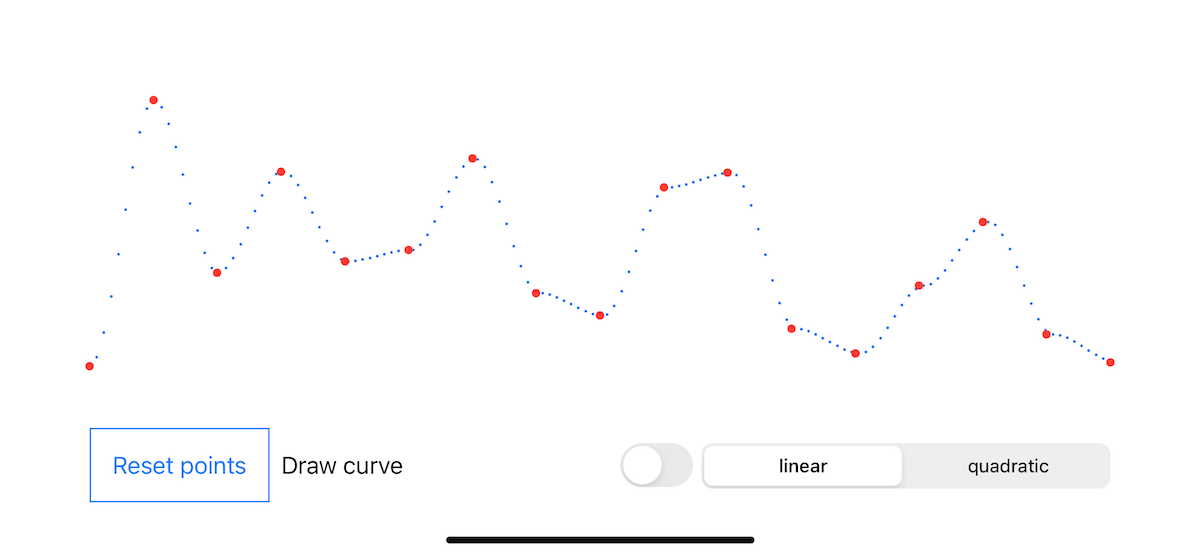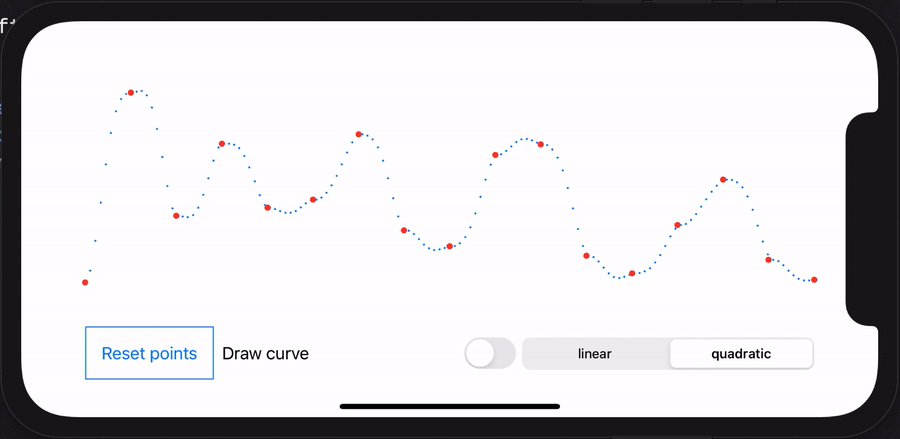I recently had to research methods to draw curves through a set of points. While interpolation wasn’t the most appropriate and efficient approach in my case, I still had some fun with the Accelerate framework (which optimizes large-scale mathematical computations).## 1. What is an interpolation?

In the mathematical field of numerical analysis, interpolation is a type of estimation, a method of constructing new data points within the range of a discrete set of known data points. (Sheppard, William Fleetwood)

For instance, let’s say you’re eating delicious pasta. Your plate contains 20 gnocchi, and you eat them continuously in a 10 min period of time. While the scenario can be managed by head ([0, 2, 4, …, 18, 20]), it’s a task that interpolation algorithms can easily achieve, whatever the numbers.

## 2. Examples to interpolate using Accelerate framework

Besides implementing the logic in simple Swift code, you can directly use the Accelerate framework which should fasten the process!

### 2.1 Simple interpolation (Gnocchi)

So let’s say we want to interpolate how the 20 gnocchi are eaten within the 10 minutes timeframe, we could write:

 ``````1 2 3 4 `````` ``````let gnocchiValues: [Double] = [0, 20] let timeIndices: [Double] = [0, 10] let result = vDSP.linearInterpolate(values: gnocchiValues, atIndices: timeIndices) ``````

The output should be `[0.0, 2.0, 4.0, 6.0, 8.0, 10.0, 12.0, 14.0, 16.0, 18.0, 20.0]`, meaning that at minute 2, 4 gnocchi should be gone.

### 2.2 Complex interpolation (CGPoint)

The previous code works fine with values that follow on another (e.g. `[1, 2, 3]`). But what about more complex inputs as set of CGPoints where y axis values go up and down? (e.g. `[(x: 1, y: 1.5), (x: 2, y: 5.0), (x: 3, y: 3.5)]`).

We could directly dive into a CGPoint example, but we’ll cover this part in the next section. For now, let’s focus on the “y” value which requires interpolation (in the case your curve is horizontal).

 `````` 1 2 3 4 5 6 7 8 9 10 11 12 `````` ``````let steps = vDSP_Length(100) // (1) let stride = vDSP_Stride(1) // (2) let values: [Float] = [1, 5, 2, 15, 20, 10, 9, 8, 15, 20] // (3) let denominator = Float(steps) / Float(values.count - 1) let control: [Float] = (0..
1. `steps` is the length of the output (number of interpolation points).
2. `stride` holds differences between indices of elements (our x axis for CGPoint).
3. `values` holds the y value of our CGPoints (as it’ll be an horizontal alignment).
4. `control` holds the fractional part to interpolate between the values.
5. `result` holds the output of the linear interpolation.

It may seem a bit harsh at first gland. I highly suggest reading the official documentation about linear interpolation functions. Note it exists for quadratic interpolation functions too.

## 3. CGPoint extension for linear interpolation

Our input vector isn’t compatible with CGPoints: we have to take care of the intermediate logic ourselves.
If you want to directly read the extension code, check this file.

Let’s create a CGPoint extension:

• Depending on the graph direction, we should use either x or y axis.
• The number of interpolated points should be dynamic.
• And let’s allow a switch between linear and quadratic interpolation.

There are two additional steps compared to the previous code:

1. Extract the “values” from the CGPoint list.
2. Construct the new CGPoint list based on the interpolation output.

Let’s put our extension inside a new file called `CGPoint+Interpolation`.

 ``````1 2 3 4 5 6 7 `````` ``````import CoreGraphics.CGGeometry import Accelerate.vecLib import simd extension Array where Element == CGPoint { } ``````

As mentionned above, we plan to a/ handle both horizontal and vertical cases, and b/ handle the linear and quadratic interpolations. For this purpose, let’s create two ENUMs:

 ``````1 2 3 4 5 6 7 `````` ``````enum InterpolationAxis: CaseIterable { case horizontal, vertical } enum InterpolationAlgorithm: CaseIterable { case linear, quadratic } ``````

Let’s keep the extension method simple:

 ``````1 2 3 4 5 6 `````` ``````func interpolated(direction: InterpolationAxis = .horizontal, algorithm: InterpolationAlgorithm = .linear, steps: Int = 1024) -> [CGPoint] { } ``````

Extracting the input vector from our CGPoint list isn’t difficult.

 ``````1 `````` ``````let values: [Float] = isHorizontal ? self.map({ Float(\$0.y) }) : self.map({ Float(\$0.x) }) ``````

However, constructing the output requires more lines as we add data instead of removing some.

 `````` 1 2 3 4 5 6 7 8 9 10 11 12 13 14 15 16 17 18 `````` ``````let pointMinimum: CGFloat = (isHorizontal ? self.first?.x : self.first?.y) ?? 0 let pointMaximum: CGFloat = (isHorizontal ? self.last?.x : self.last?.y) ?? 0 let pointStep: CGFloat = (pointMaximum - pointMinimum) / CGFloat(steps - 1) if isHorizontal == true { return result.enumerated().map { (index, value) -> CGPoint in CGPoint( x: pointMinimum + (CGFloat(index) * pointStep), y: CGFloat(value) ) } } else { return result.enumerated().map { (index, value) -> CGPoint in CGPoint( x: CGFloat(value), y: pointMinimum + (CGFloat(index) * pointStep) ) } } ``````

Then you merge this with the logic we wrote a bit earlier, and voilà.

You can read the full code in the GitHub project, and specifically here.

## 4. Wrapping the fun inside a SwiftUI project

I spent some time having fun with SwiftUI. You can download the Xcode project and play with it. Check the animation below as a small demo.• The red points are randomly generated.
• The blue points are generated from the interpolation.
• The blue line is drawn from the interpolation points.
• You can switch between linear and quadratic algorithm.

Special thanks to Axel Le Pennec for mentioning the interpolation (Accelerate) methods to me.